# RD Sharma Solutions For Class 7 Maths Chapter 15 Properties of Triangles Exercise 15.1

RD Sharma Solutions for Class 7 Maths Exercise 15.1 of Chapter 15 Properties of Triangles in PDF are available here. Learners can download the PDF from the provided links. RD Sharma Solutions for Class 7 provides solutions for all topics covered under this chapter. In this exercise, students will learn about the basic concepts related to triangles and their properties. Several topics of this exercise are listed below:

• Definition and meaning of triangles
• Interior and exterior angle of the triangle
• Triangular region
• Types of the triangle by considering the lengths of their sides

## Download the PDF of RD Sharma Solutions For Class 7 Maths Chapter 15 – Properties of Triangles Exercise 15.1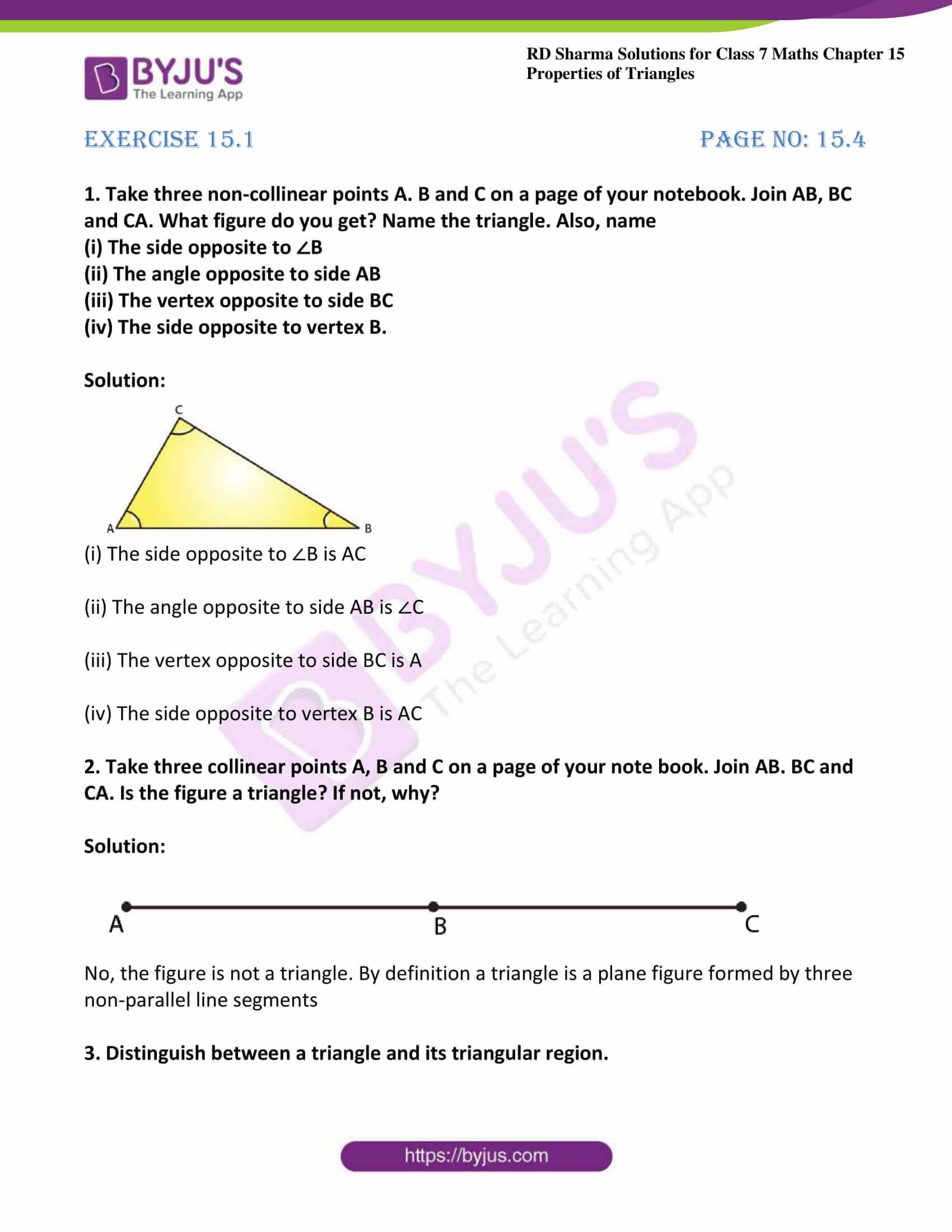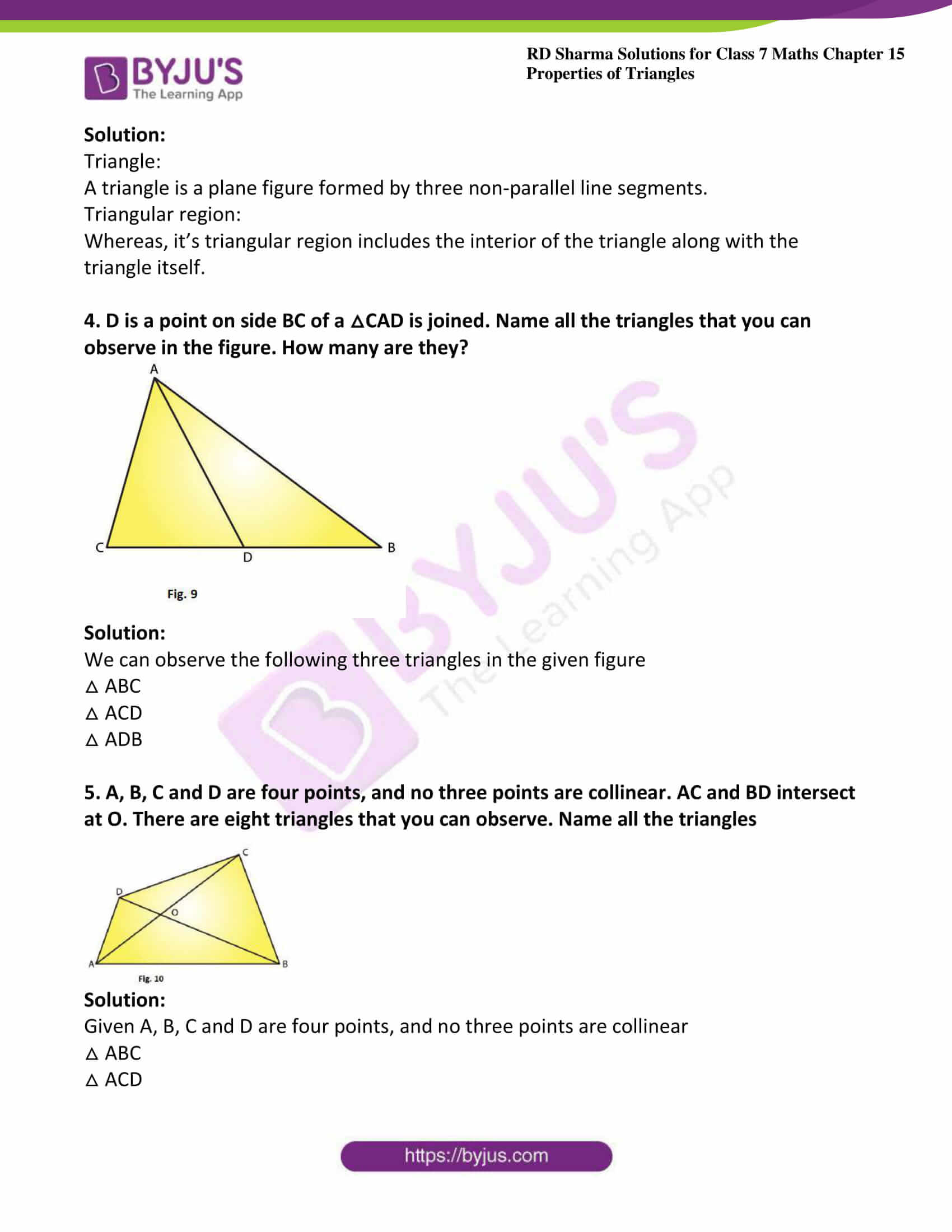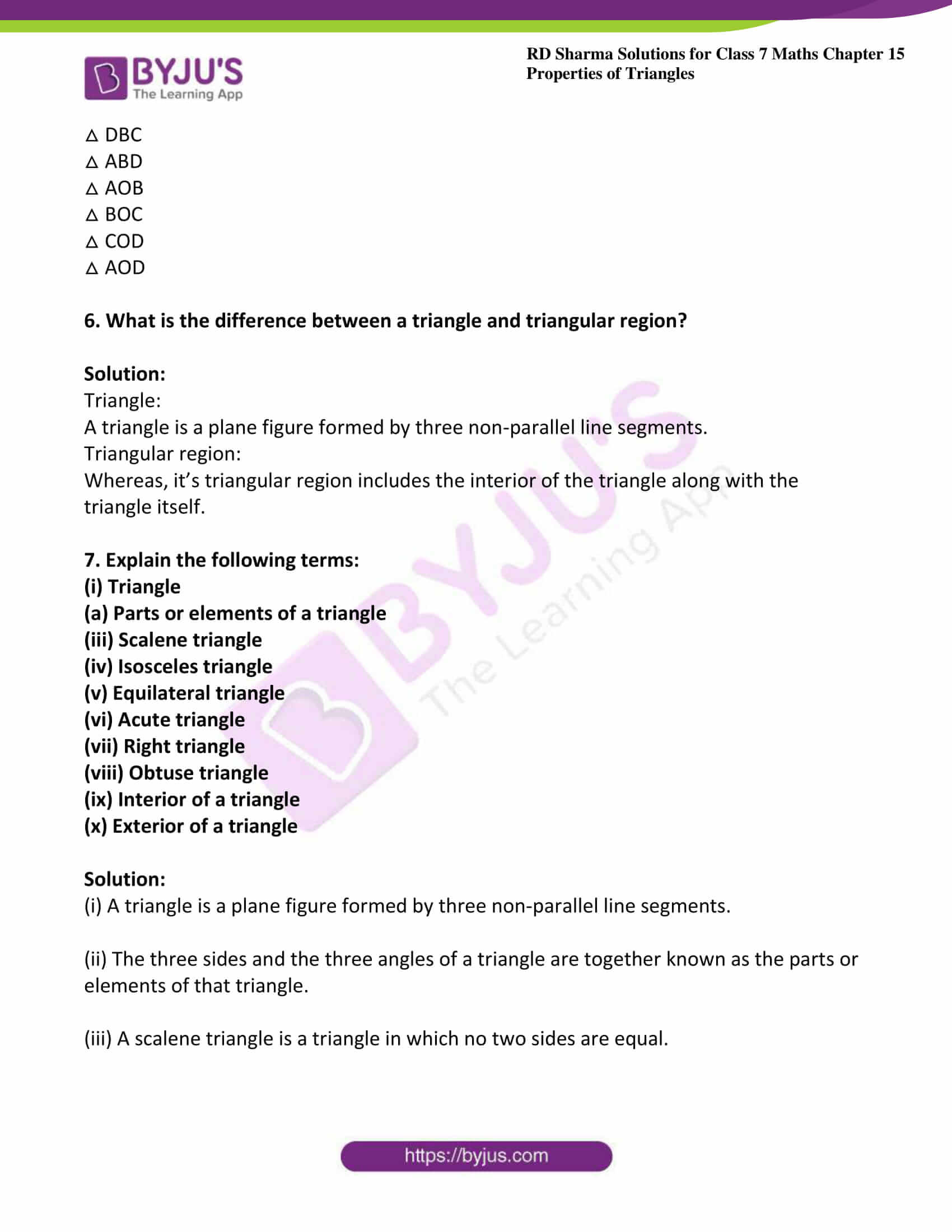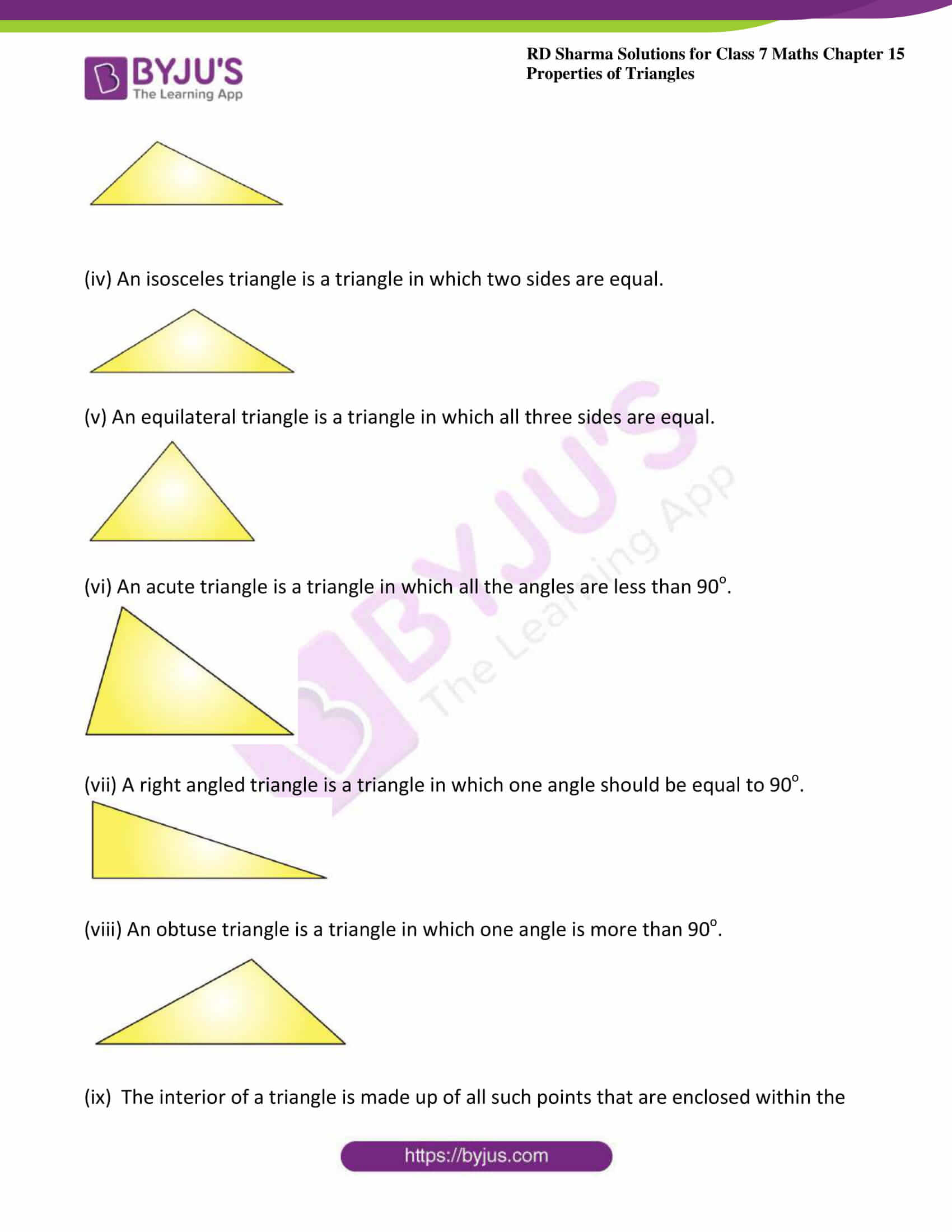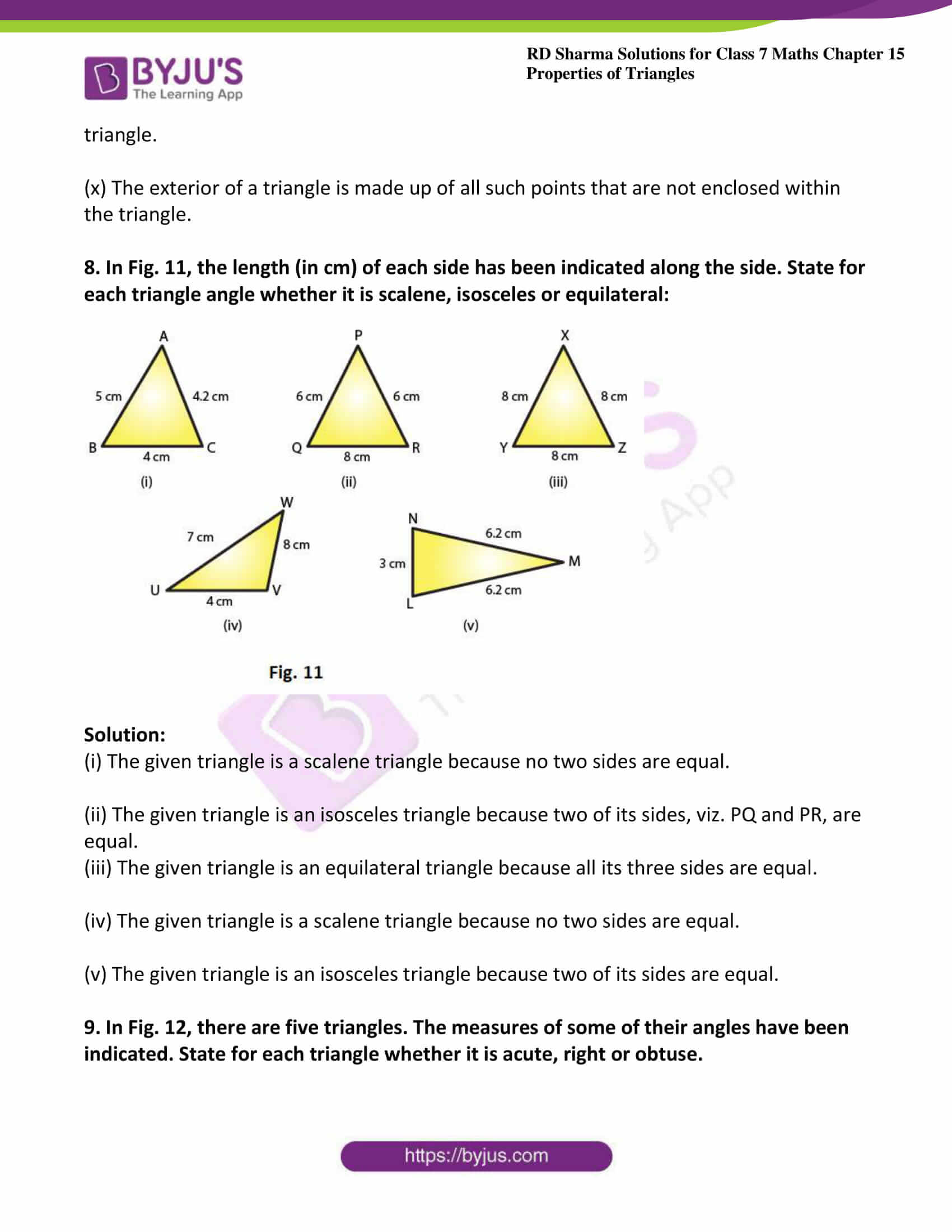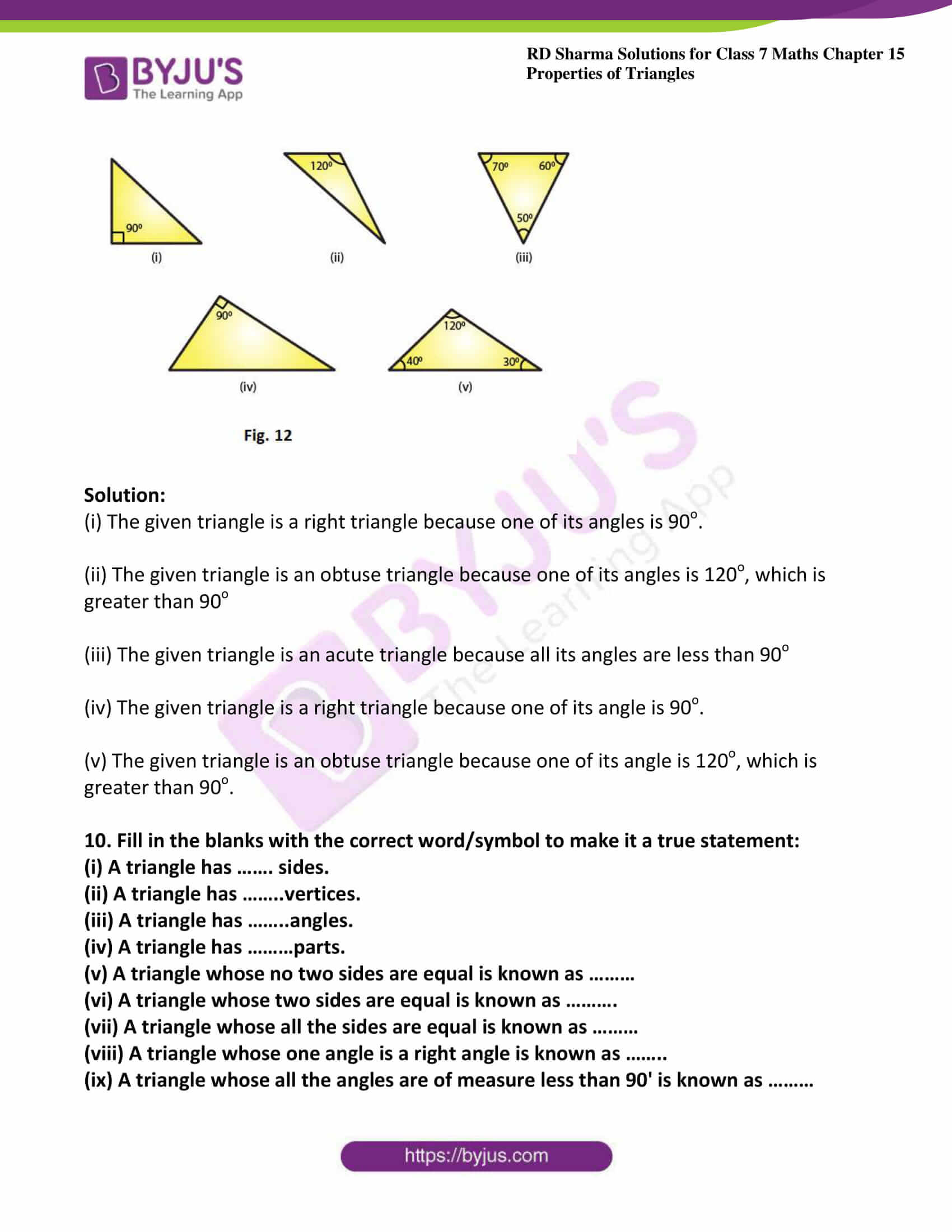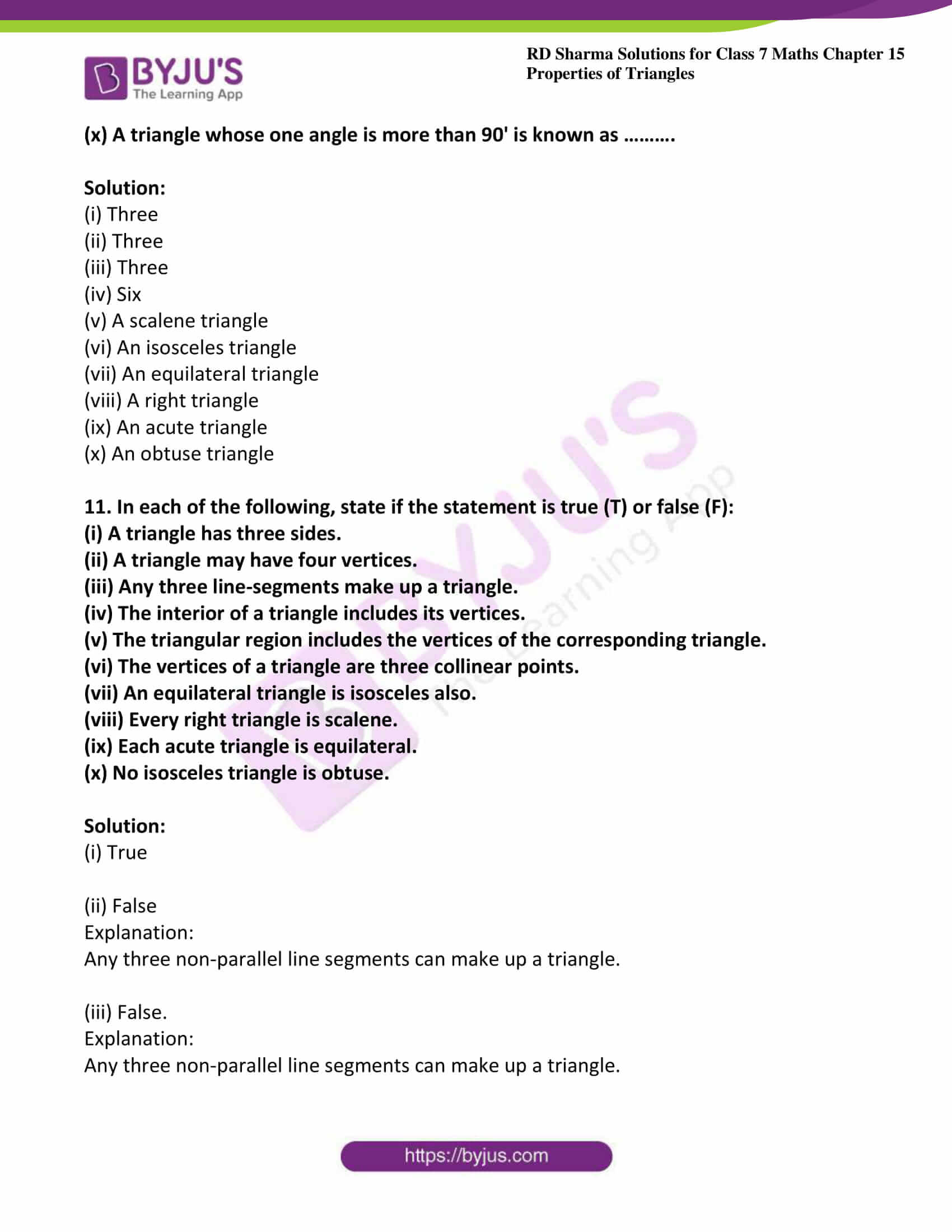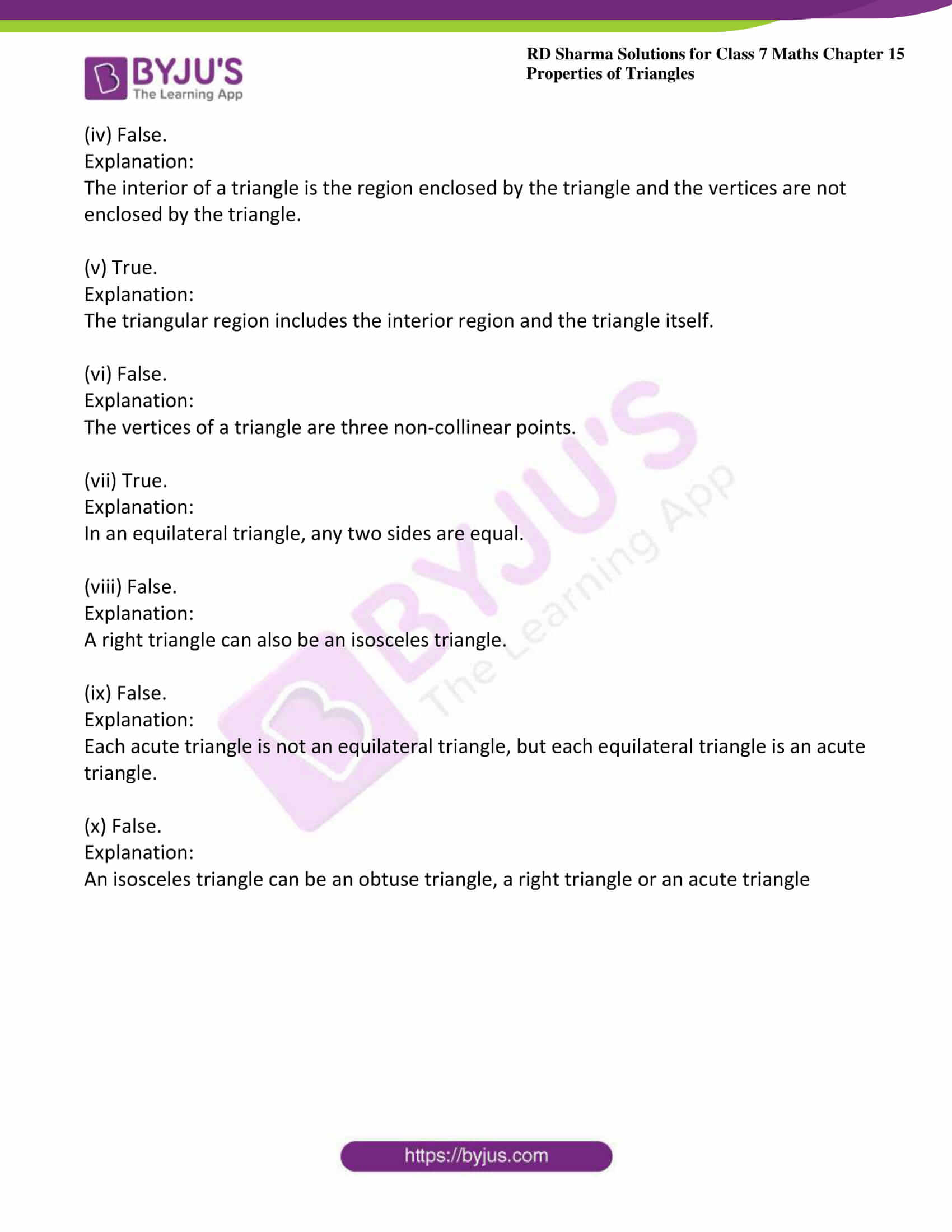### Access answers to Maths RD Sharma Solutions For Class 7 Chapter 15 – Properties of Triangles Exercise 15.1

Exercise 15.1 Page No: 15.4

1. Take three non-collinear points A. B and C on a page of your notebook. Join AB, BC and CA. What figure do you get? Name the triangle. Also, name

(i) The side opposite to ∠B

(ii) The angle opposite to side AB

(iii) The vertex opposite to side BC

(iv) The side opposite to vertex B.

Solution: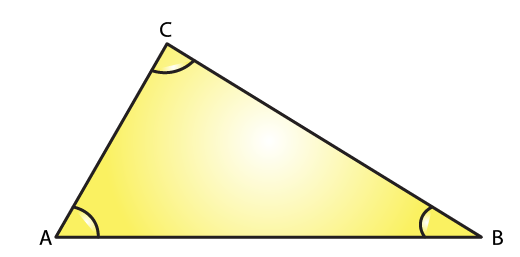(i) The side opposite to ∠B is AC

(ii) The angle opposite to side AB is ∠C

(iii) The vertex opposite to side BC is A

(iv) The side opposite to vertex B is AC

2. Take three collinear points A, B and C on a page of your note book. Join AB. BC and CA. Is the figure a triangle? If not, why?

Solution: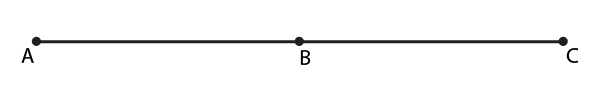No, the figure is not a triangle. By definition a triangle is a plane figure formed by three non-parallel line segments

3. Distinguish between a triangle and its triangular region.

Solution:

Triangle:

A triangle is a plane figure formed by three non-parallel line segments.

Triangular region:

Whereas, it’s triangular region includes the interior of the triangle along with the triangle itself.

4. D is a point on side BC of a △CAD is joined. Name all the triangles that you can observe in the figure. How many are they?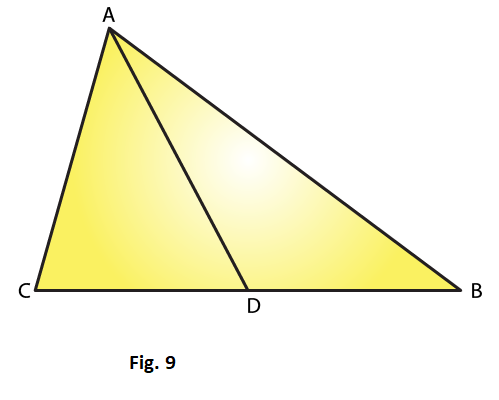Solution:

We can observe the following three triangles in the given figure

△ ABC

△ ACD

5. A, B, C and D are four points, and no three points are collinear. AC and BD intersect at O. There are eight triangles that you can observe. Name all the triangles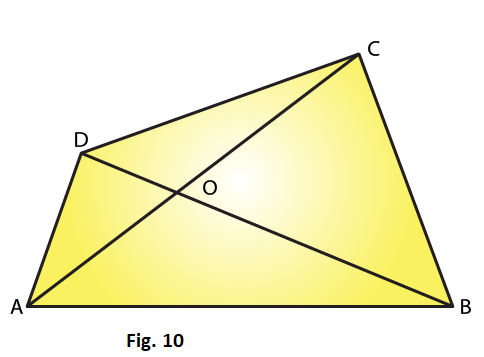Solution:

Given A, B, C and D are four points, and no three points are collinear

△ ABC

△ ACD

△ DBC

△ ABD

△ AOB

△ BOC

△ COD

△ AOD

6. What is the difference between a triangle and triangular region?

Solution:

Triangle:

A triangle is a plane figure formed by three non-parallel line segments.

Triangular region:

Whereas, it’s triangular region includes the interior of the triangle along with the triangle itself.

7. Explain the following terms:

(i) Triangle

(a) Parts or elements of a triangle

(iii) Scalene triangle

(iv) Isosceles triangle

(v) Equilateral triangle

(vi) Acute triangle

(vii) Right triangle

(viii) Obtuse triangle

(ix) Interior of a triangle

(x) Exterior of a triangle

Solution:

(i) A triangle is a plane figure formed by three non-parallel line segments.

(ii) The three sides and the three angles of a triangle are together known as the parts or elements of that triangle.

(iii) A scalene triangle is a triangle in which no two sides are equal.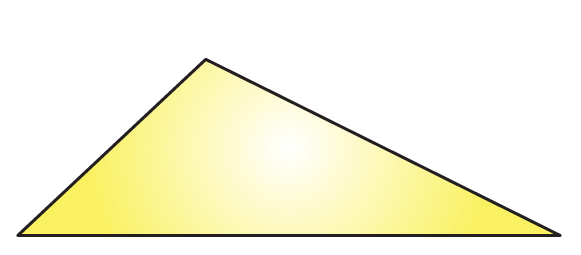(iv) An isosceles triangle is a triangle in which two sides are equal.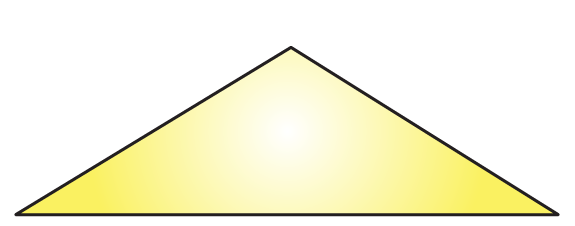(v) An equilateral triangle is a triangle in which all three sides are equal.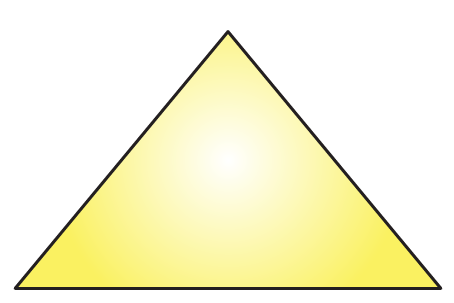(vi) An acute triangle is a triangle in which all the angles are less than 90o.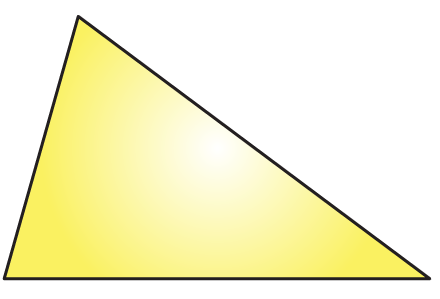(vii) A right angled triangle is a triangle in which one angle should be equal to 90o.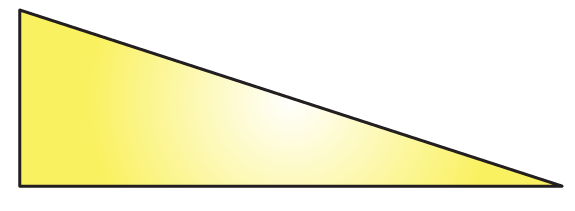(viii) An obtuse triangle is a triangle in which one angle is more than 90o.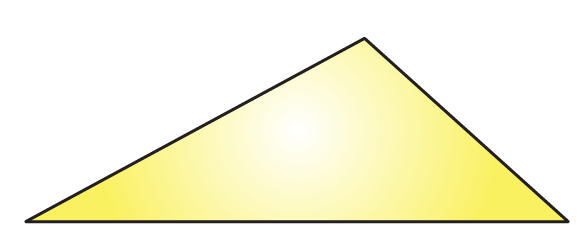(ix)  The interior of a triangle is made up of all such points that are enclosed within the triangle.

(x) The exterior of a triangle is made up of all such points that are not enclosed within the triangle.

8. In Fig. 11, the length (in cm) of each side has been indicated along the side. State for each triangle angle whether it is scalene, isosceles or equilateral: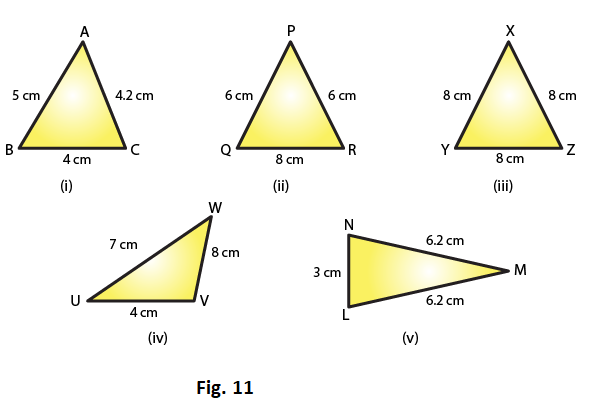Solution:

(i) The given triangle is a scalene triangle because no two sides are equal.

(ii) The given triangle is an isosceles triangle because two of its sides, viz. PQ and PR, are equal.

(iii) The given triangle is an equilateral triangle because all its three sides are equal.

(iv) The given triangle is a scalene triangle because no two sides are equal.

(v) The given triangle is an isosceles triangle because two of its sides are equal.

9. In Fig. 12, there are five triangles. The measures of some of their angles have been indicated. State for each triangle whether it is acute, right or obtuse.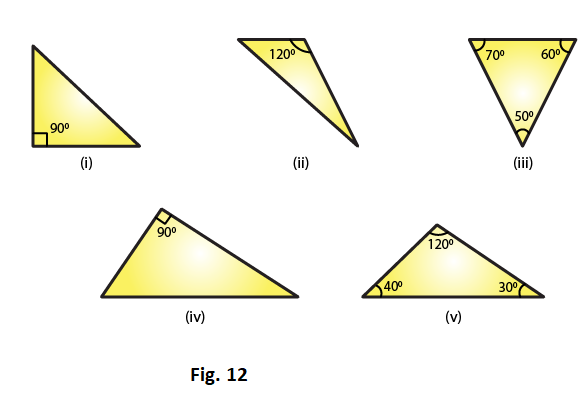Solution:

(i) The given triangle is a right triangle because one of its angles is 90o.

(ii) The given triangle is an obtuse triangle because one of its angles is 120o, which is greater than 90o

(iii) The given triangle is an acute triangle because all its angles are less than 90o

(iv) The given triangle is a right triangle because one of its angle is 90o.

(v) The given triangle is an obtuse triangle because one of its angle is 120o, which is greater than 90o.

10. Fill in the blanks with the correct word/symbol to make it a true statement:

(i) A triangle has ……. sides.

(ii) A triangle has ……..vertices.

(iii) A triangle has ……..angles.

(iv) A triangle has ………parts.

(v) A triangle whose no two sides are equal is known as ………

(vi) A triangle whose two sides are equal is known as ……….

(vii) A triangle whose all the sides are equal is known as ………

(viii) A triangle whose one angle is a right angle is known as ……..

(ix) A triangle whose all the angles are of measure less than 90′ is known as ………

(x) A triangle whose one angle is more than 90′ is known as ……….

Solution:

(i) Three

(ii) Three

(iii) Three

(iv) Six

(v) A scalene triangle

(vi) An isosceles triangle

(vii) An equilateral triangle

(viii) A right triangle

(ix) An acute triangle

(x) An obtuse triangle

11. In each of the following, state if the statement is true (T) or false (F):

(i) A triangle has three sides.

(ii) A triangle may have four vertices.

(iii) Any three line-segments make up a triangle.

(iv) The interior of a triangle includes its vertices.

(v) The triangular region includes the vertices of the corresponding triangle.

(vi) The vertices of a triangle are three collinear points.

(vii) An equilateral triangle is isosceles also.

(viii) Every right triangle is scalene.

(ix) Each acute triangle is equilateral.

(x) No isosceles triangle is obtuse.

Solution:

(i) True

(ii) False

Explanation:

Any three non-parallel line segments can make up a triangle.

(iii) False.

Explanation:

Any three non-parallel line segments can make up a triangle.

(iv) False.

Explanation:

The interior of a triangle is the region enclosed by the triangle and the vertices are not enclosed by the triangle.

(v) True.

Explanation:

The triangular region includes the interior region and the triangle itself.

(vi) False.

Explanation:

The vertices of a triangle are three non-collinear points.

(vii) True.

Explanation:

In an equilateral triangle, any two sides are equal.

(viii) False.

Explanation:

A right triangle can also be an isosceles triangle.

(ix) False.

Explanation:

Each acute triangle is not an equilateral triangle, but each equilateral triangle is an acute triangle.

(x) False.

Explanation:

An isosceles triangle can be an obtuse triangle, a right triangle or an acute triangle